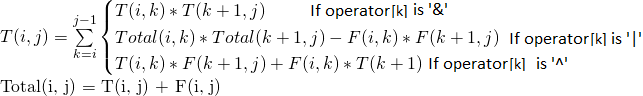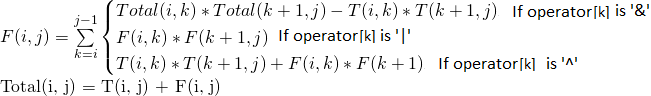# Boolean Parenthesization Problem | DP-37

Given a boolean expression with following symbols.

Symbols
'T' ---> true
'F' ---> false 

And following operators filled between symbols

Operators
&   ---> boolean AND
|   ---> boolean OR
^   ---> boolean XOR 

Count the number of ways we can parenthesize the expression so that the value of expression evaluates to true.

Let the input be in form of two arrays one contains the symbols (T and F) in order and other contains operators (&, | and ^}

Examples:

Input: symbol[]    = {T, F, T}
operator[]  = {^, &}
Output: 2
The given expression is "T ^ F & T", it evaluates true
in two ways "((T ^ F) & T)" and "(T ^ (F & T))"

Input: symbol[]    = {T, F, F}
operator[]  = {^, |}
Output: 2
The given expression is "T ^ F | F", it evaluates true
in two ways "( (T ^ F) | F )" and "( T ^ (F | F) )".

Input: symbol[]    = {T, T, F, T}
operator[]  = {|, &, ^}
Output: 4
The given expression is "T | T & F ^ T", it evaluates true
in 4 ways ((T|T)&(F^T)), (T|(T&(F^T))), (((T|T)&F)^T)
and (T|((T&F)^T)).


## Recommended: Please solve it on “PRACTICE” first, before moving on to the solution.

Solution:
Let T(i, j) represents the number of ways to parenthesize the symbols between i and j (both inclusive) such that the subexpression between i and j evaluates to true.<!––>

Let F(i, j) represents the number of ways to parenthesize the symbols between i and j (both inclusive) such that the subexpression between i and j evaluates to false.<!—–>
Base Cases:

T(i, i) = 1 if symbol[i] = 'T'
T(i, i) = 0 if symbol[i] = 'F'

F(i, i) = 1 if symbol[i] = 'F'
F(i, i) = 0 if symbol[i] = 'T'

If we draw recursion tree of above recursive solution, we can observe that it many overlapping subproblems. Like other dynamic programming problems, it can be solved by filling a table in bottom up manner. Following is C++ implementation of dynamic programming solution.

## C++

 #include  #include  using namespace std;     // Returns count of all possible parenthesizations that lead to  // result true for a boolean expression with symbols like true  // and false and operators like &, | and ^ filled between symbols  int countParenth(char symb[], char oper[], int n)  {      int F[n][n], T[n][n];         // Fill diaginal entries first      // All diagonal entries in T[i][i] are 1 if symbol[i]      // is T (true).  Similarly, all F[i][i] entries are 1 if      // symbol[i] is F (False)      for (int i = 0; i < n; i++)      {          F[i][i] = (symb[i] == 'F')? 1: 0;          T[i][i] = (symb[i] == 'T')? 1: 0;      }         // Now fill T[i][i+1], T[i][i+2], T[i][i+3]... in order      // And F[i][i+1], F[i][i+2], F[i][i+3]... in order      for (int gap=1; gap

## Java

 class GFG   {             // Returns count of all possible       // parenthesizations that lead to      // result true for a boolean       // expression with symbols like true      // and false and operators like &, |       // and ^ filled between symbols      static int countParenth(char symb[],                       char oper[], int n)          {          int F[][] = new int[n][n];          int T[][] = new int[n][n];             // Fill diaginal entries first          // All diagonal entries in T[i][i]           // are 1 if symbol[i] is T (true).           // Similarly, all F[i][i] entries           // are 1 if symbol[i] is F (False)          for (int i = 0; i < n; i++)           {              F[i][i] = (symb[i] == 'F') ? 1 : 0;              T[i][i] = (symb[i] == 'T') ? 1 : 0;          }             // Now fill T[i][i+1], T[i][i+2],           // T[i][i+3]... in order And F[i][i+1],          // F[i][i+2], F[i][i+3]... in order          for (int gap = 1; gap < n; ++gap)          {              for (int i = 0, j = gap; j < n; ++i, ++j)               {                  T[i][j] = F[i][j] = 0;                  for (int g = 0; g < gap; g++)                   {                      // Find place of parenthesization                       // using current value of gap                      int k = i + g;                         // Store Total[i][k] and Total[k+1][j]                      int tik = T[i][k] + F[i][k];                      int tkj = T[k + 1][j] + F[k + 1][j];                         // Follow the recursive formulas                       // according to the current operator                      if (oper[k] == '&')                       {                          T[i][j] += T[i][k] * T[k + 1][j];                          F[i][j] += (tik * tkj - T[i][k] * T[k + 1][j]);                      }                      if (oper[k] == '|')                       {                          F[i][j] += F[i][k] * F[k + 1][j];                          T[i][j] += (tik * tkj - F[i][k] * F[k + 1][j]);                      }                      if (oper[k] == '^')                      {                          T[i][j] += F[i][k] * T[k + 1][j] +                                       T[i][k] * F[k + 1][j];                          F[i][j] += T[i][k] * T[k + 1][j] +                                       F[i][k] * F[k + 1][j];                      }                  }              }          }          return T[n - 1];      }         // Driver code      public static void main(String[] args)       {          char symbols[] = "TTFT".toCharArray();          char operators[] = "|&^".toCharArray();          int n = symbols.length;             // There are 4 ways          // ((T|T)&(F^T)), (T|(T&(F^T))),           // (((T|T)&F)^T) and (T|((T&F)^T))          System.out.println(countParenth(symbols,                              operators, n));      }  }     // This code has been contributed   // by 29AjayKumar 

## Python3

 # Returns count of all possible   # parenthesizations that lead to   # result true for a boolean   # expression with symbols like    # true and false and operators   # like &, | and ^ filled between symbols   def countParenth(symb, oper, n):      F = [[0 for i in range(n + 1)]               for i in range(n + 1)]      T = [[0 for i in range(n + 1)]               for i in range(n + 1)]                     # Fill diaginal entries first       # All diagonal entries in       # T[i][i] are 1 if symbol[i]       # is T (true). Similarly, all      # F[i][i] entries are 1 if       # symbol[i] is F (False)       for i in range(n):          if symb[i] == 'F':              F[i][i] = 1         else:              F[i][i] = 0            if symb[i] == 'T':              T[i][i] = 1         else:              T[i][i] = 0                    # Now fill T[i][i+1], T[i][i+2],       # T[i][i+3]... in order And       # F[i][i+1], F[i][i+2],       # F[i][i+3]... in order      for gap in range(1, n):          i = 0         for j in range(gap, n):               T[i][j] = F[i][j] = 0             for g in range(gap):                                     # Find place of parenthesization                   # using current value of gap                  k = i + g                                     # Store Total[i][k] and Total[k+1][j]                   tik = T[i][k] + F[i][k];                   tkj = T[k + 1][j] + F[k + 1][j];                                     # Follow the recursive formulas                   # according to the current operator                  if oper[k] == '&':                      T[i][j] += T[i][k] * T[k + 1][j]                      F[i][j] += (tik * tkj - T[i][k] *                                              T[k + 1][j])                  if oper[k] == '|':                      F[i][j] += F[i][k] * F[k + 1][j]                      T[i][j] += (tik * tkj - F[i][k] *                                              F[k + 1][j])                  if oper[k]=='^':                      T[i][j] += (F[i][k] * T[k + 1][j] +                                  T[i][k] * F[k + 1][j])                       F[i][j] += (T[i][k] * T[k + 1][j] +                                  F[i][k] * F[k + 1][j])              i += 1     return T[n - 1]          # Driver Code  symbols = "TTFT" operators = "|&^" n = len(symbols)     # There are 4 ways   # ((T|T)&(F^T)), (T|(T&(F^T))),   # (((T|T)&F)^T) and (T|((T&F)^T))   print(countParenth(symbols, operators, n))     # This code is contributed by   # sahil shelangia 

## C#

 // C# program of above approach  using System;     class GFG   {             // Returns count of all possible       // parenthesizations that lead to      // result true for a boolean       // expression with symbols like true      // and false and operators like &, |       // and ^ filled between symbols      static int countParenth(char []symb,                       char []oper, int n)       {          int [,]F = new int[n, n];          int [,]T = new int[n, n];             // Fill diaginal entries first          // All diagonal entries in T[i,i]           // are 1 if symbol[i] is T (true).           // Similarly, all F[i,i] entries           // are 1 if symbol[i] is F (False)          for (int i = 0; i < n; i++)           {              F[i,i] = (symb[i] == 'F') ? 1 : 0;              T[i,i] = (symb[i] == 'T') ? 1 : 0;          }             // Now fill T[i,i+1], T[i,i+2],           // T[i,i+3]... in order And F[i,i+1],          // F[i,i+2], F[i,i+3]... in order          for (int gap = 1; gap < n; ++gap)          {              for (int i = 0, j = gap; j < n; ++i, ++j)               {                  T[i, j] = F[i, j] = 0;                  for (int g = 0; g < gap; g++)                   {                      // Find place of parenthesization                       // using current value of gap                      int k = i + g;                         // Store Total[i,k] and Total[k+1,j]                      int tik = T[i, k] + F[i, k];                      int tkj = T[k + 1, j] + F[k + 1, j];                         // Follow the recursive formulas                       // according to the current operator                      if (oper[k] == '&')                       {                          T[i, j] += T[i, k] * T[k + 1, j];                          F[i, j] += (tik * tkj - T[i, k] * T[k + 1, j]);                      }                      if (oper[k] == '|')                       {                          F[i,j] += F[i, k] * F[k + 1, j];                          T[i,j] += (tik * tkj - F[i, k] * F[k + 1, j]);                      }                      if (oper[k] == '^')                      {                          T[i, j] += F[i, k] * T[k + 1, j] +                                       T[i, k] * F[k + 1, j];                          F[i, j] += T[i, k] * T[k + 1, j] +                                       F[i, k] * F[k + 1, j];                      }                  }              }          }          return T[0,n - 1];      }         // Driver code      public static void Main()       {          char []symbols = "TTFT".ToCharArray();          char []operators = "|&^".ToCharArray();          int n = symbols.Length;             // There are 4 ways          // ((T|T)&(F^T)), (T|(T&(F^T))),           // (((T|T)&F)^T) and (T|((T&F)^T))          Console.WriteLine(countParenth(symbols,                              operators, n));      }  }     /* This code contributed by PrinciRaj1992 */

Output:

4`

Time Complexity:
O(n3)
Auxiliary Space: O(n2)